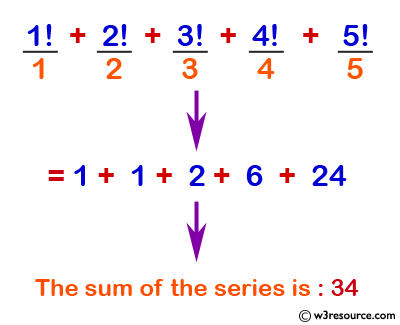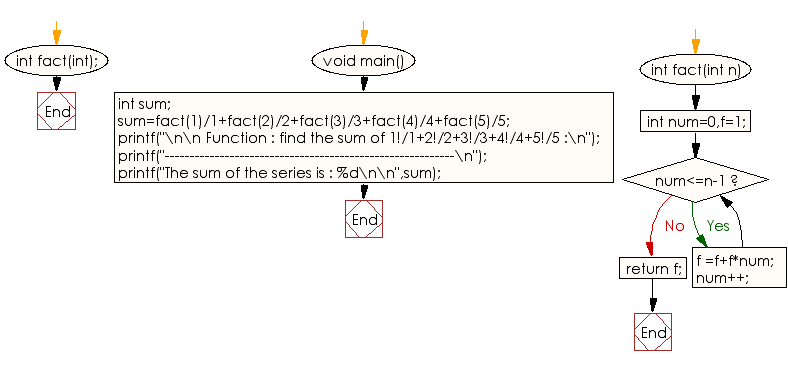﻿ C Program: Find the sum of specified series - w3resource

# C Exercises: Find the sum of specified series

## C Function : Exercise-5 with Solution

Write a program in C to find the sum of the series 1!/1+2!/2+3!/3+4!/4+5!/5 using the function.

Pictorial Presentation:Sample Solution:

C Code:

``````#include <stdio.h>

int fact(int);
void main()
{
int sum;
sum=fact(1)/1+fact(2)/2+fact(3)/3+fact(4)/4+fact(5)/5;
printf("\n\n Function : find the sum of 1!/1+2!/2+3!/3+4!/4+5!/5 :\n");
printf("----------------------------------------------------------\n");
printf("The sum of the series is : %d\n\n",sum);
}

int fact(int n)
{
int num=0,f=1;
while(num<=n-1)
{
f =f+f*num;
num++;
}
return f;
}
```
```

Sample Output:

``` Function : find the sum of 1!/1+2!/2+3!/3+4!/4+5!/5 :
----------------------------------------------------------
The sum of the series is : 34
```

Explanation:

```int fact(int n) {
int num = 0, f = 1;
while (num <= n - 1) {
f = f + f * num;
num++;
}
return f;
}
```

In the above code the function 'fact' takes a single argument 'n' (type int). It computes the factorial of the input number 'n' using a while loop. The function initializes two local integer variables, 'num' and 'f', to 0 and 1 respectively. It then enters a loop that iterates n-1 times, incrementing num by 1 in each iteration. In each iteration of the loop, the value of f is updated by multiplying it with num incremented by 1. This computes the factorial of 'n' and returns the result.

Time complexity and space complexity:

The time complexity of the function 'fact' is O(n), where 'n' is the input number, as the loop in the function iterates n-1 times.

The space complexity of this function is O(1), as it only uses a fixed amount of memory to store the two integer variables 'num' and 'f'.

Flowchart:C Programming Code Editor:

What is the difficulty level of this exercise?

Test your Programming skills with w3resource's quiz.

﻿